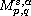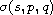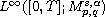Electron. J. Differential Equations, Vol. 2016 (2016), No. 338, pp. 1-12.

### Critical exponent for the heat equation in-modulation spaces Wang Zheng, Huang Qiang, Bu Rui

Abstract:
In this article, we propose a method for finding the critical exponent for heat equations in-modulation space. We define an index, and use it to determine the critical exponent of the heat equation. Then we use this exponent to describe well and ill-posedness of the heat equation in. In some special case our conclusions are sharp. Furthermore, our method may be applied to other evolution equations.

Submitted January 27, 2016. Published December 30, 2016.
Math Subject Classifications: 35A01, 35A02, 42B37.
Key Words: alpha-modulation space; heat equation; critical exponent.

Show me the PDF file (257 KB), TEX file for this article.Wang Zheng School of Mathematical Sciences Zhejiang University Hangzhou 310027, China email: wangzheng10.17@163.com Huang Qiang School of Mathematical Sciences Zhejiang University Hangzhou 310027, China email: huangqiang0704@163.com Bu Rui Department of Mathematics Qingdao University of Science and Technology Qingdao 266061, China email: burui0@163.com